# Home

Henry Adams edited this page Aug 9, 2018 · 127 revisions

# Introduction

This tutorial accompanies the TRIPODS Summer Bootcamp: Topology and Machine Learning, https://icerm.brown.edu/tripods/tri18-2-tml/. It was written by Henry Adams, Melissa McGuirl, and Yitzchak Solomon. It relies on code from scikit-learn (http://scikit-learn.org/stable/), Ripser (https://github.com/Ripser/ripser), and a variety of other places, referenced throughout the text.

If you code up a cool related example, please let us know and we may add it to this repository!

Here is a link to Isaac's recommended restaurants! https://docs.google.com/document/d/1c1hfA6Wlp5qIpu3G1Q-zyHq1jOLHCTyHsex17_UHKg4/edit?usp=sharing

# Python

The code in this repository is written in Python.

Installing and running new code is annoying, especially if it is in a language (perhaps Python) that is unfamiliar to you. Nevertheless, we believe that it is extremely important for all practitioners of machine learning to have some exposure to Python. For this reason, the time you spend getting python running on your machine is time well spent, even though this can feel like a frustrating investment of time.

If you don't yet have Python, and if you are a PC user, then we recommend installing Anaconda (https://www.anaconda.com/download/#macos). If you don't yet have Python, and if you are a Mac user, then we recommend installing Python 2.7.15 at (https://www.python.org/downloads/release/python-2715/). We expect the code to work with an existing version of Python that you may happen to have already.

# Machine learning

The code in this section is taken and modified from http://scikit-learn.org/stable/auto_examples/classification/plot_classifier_comparison.html. For further examples of machine learning with scikit-learn, see http://scikit-learn.org/stable/tutorial/basic/tutorial.html and http://scikit-learn.org/stable/user_guide.html.

## Classifying

Classifying is a supervised task in machine learning. Given a collection of labeled data points, how do we build a classifier such that when given a new unlabeled data point, we can predict its label with high accuracy? There are a wide variety of classifying techniques.

Open a terminal on your machine, and change directories to the `Top-ML/machine-learning` folder.

In the terminal, try typing the following command.

``````python classifier_compare.py
``````

You will get a long list of warnings; please ignore those warnings. The code takes about a second to run. You should get something like the following image as output!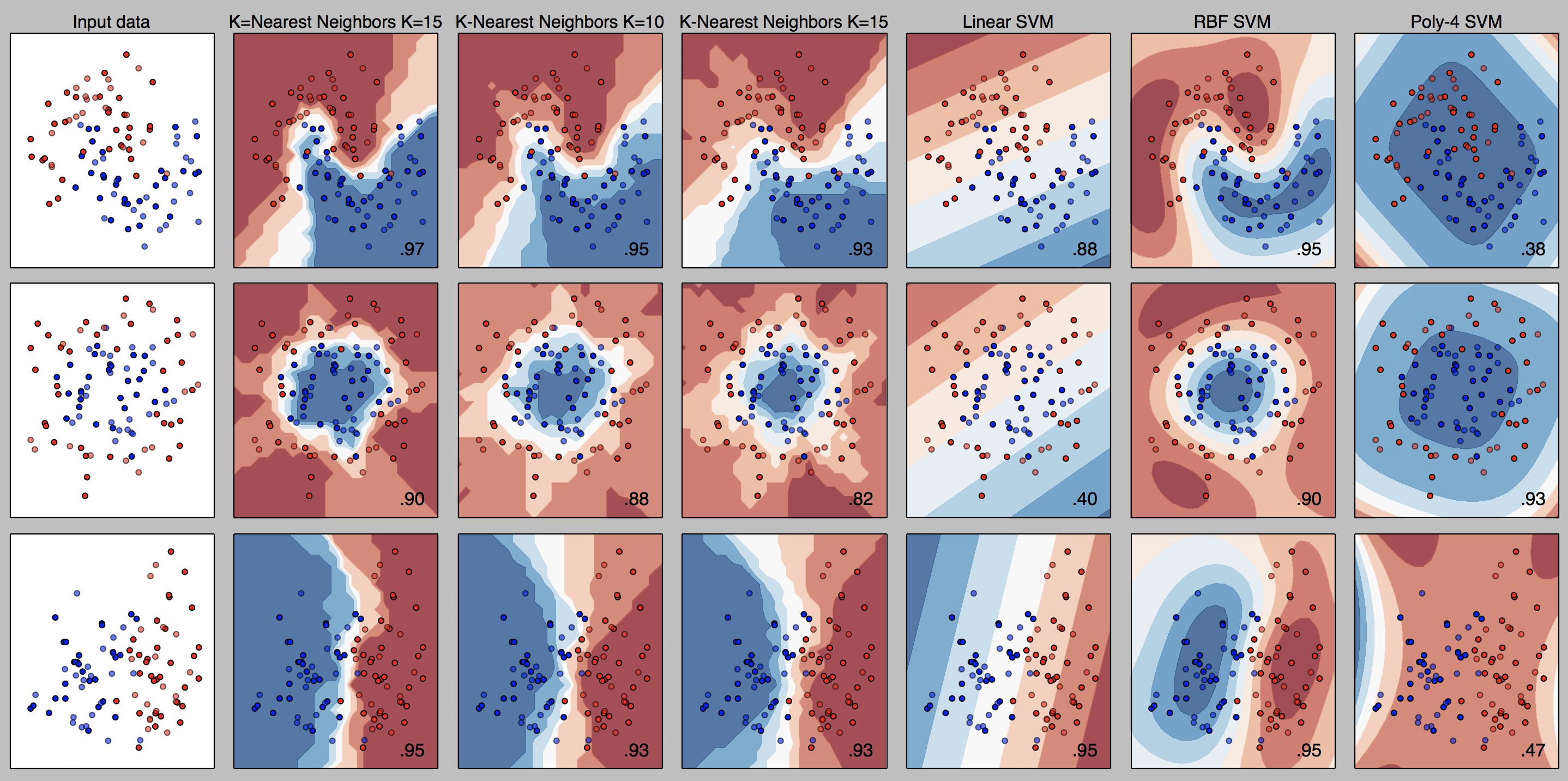The first column is the input data, the next three columns show a K nearest neighbors classification for different values of K, and the final three columns show Support Vector Machine (SVM) classifiers, with linear, radial, and polynomial kernels.

## Clustering

Clustering is an unsupervised method in machine learning. Given a collection of (unlabeled) data, how do we best divide the data into clusters, where data points within the same cluster are similar to each other? There are a wide variety of clustering techniques, a few of which we visualize now.

Still in the `Top-ML/machine-learning` folder, try typing the following command into the terminal.

``````python cluster_compare.py
``````

You will get a long list of warnings; please ignore those warnings. The code takes less than 30 seconds to run. You should get something like the following image as output!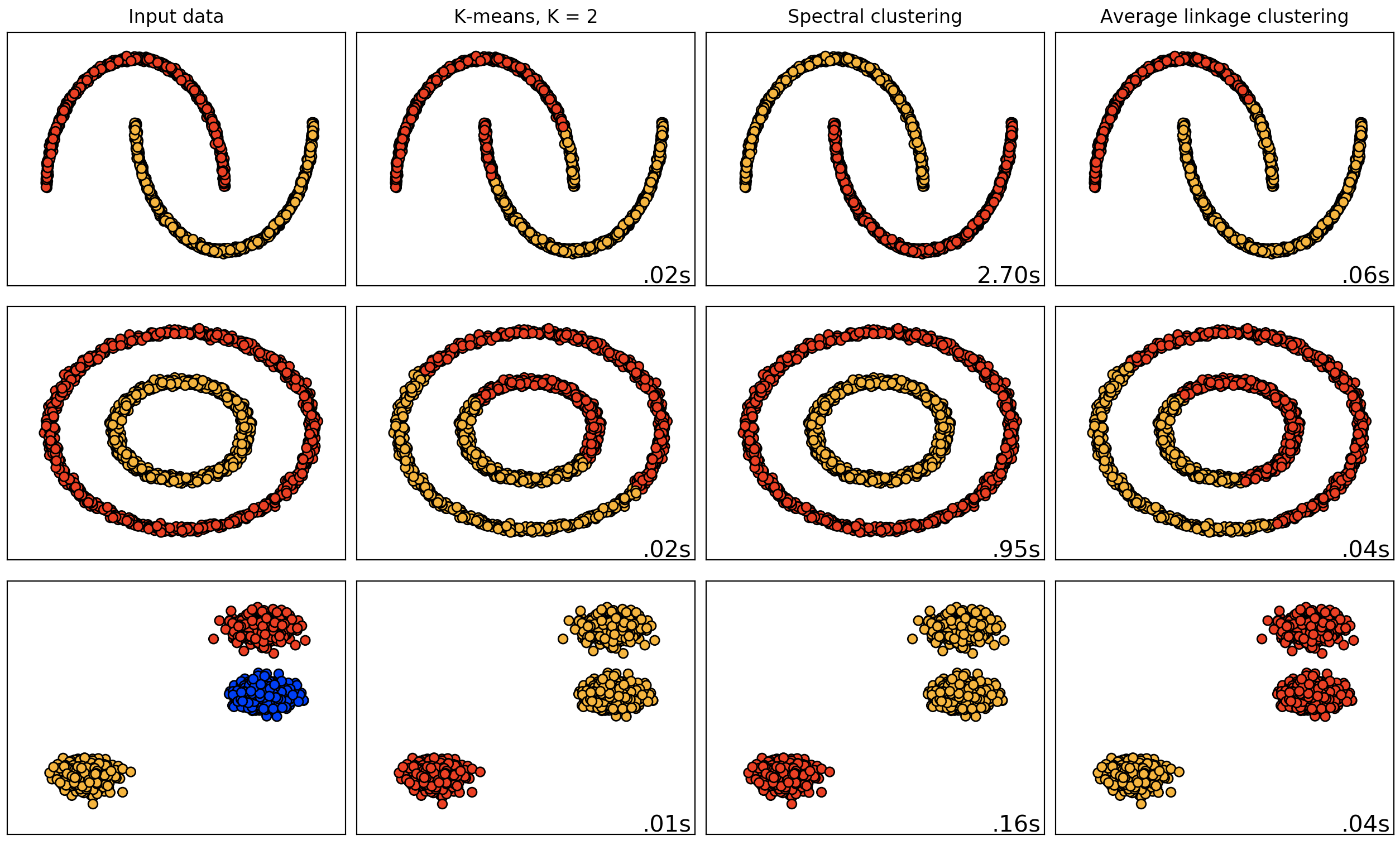The first column is the input data, the second column is the output from a K means clustering, the third column is the output from a spectral clustering algorithm, and the third column is the output from an average linkage clustering algorithm.

If you're adventurous, try changing the number of clusters (on lines 39-40 of `cluster_compare.py` from 2 to 3. After doing so, you should instead get output such as the following.

``````python cluster_compare.py
``````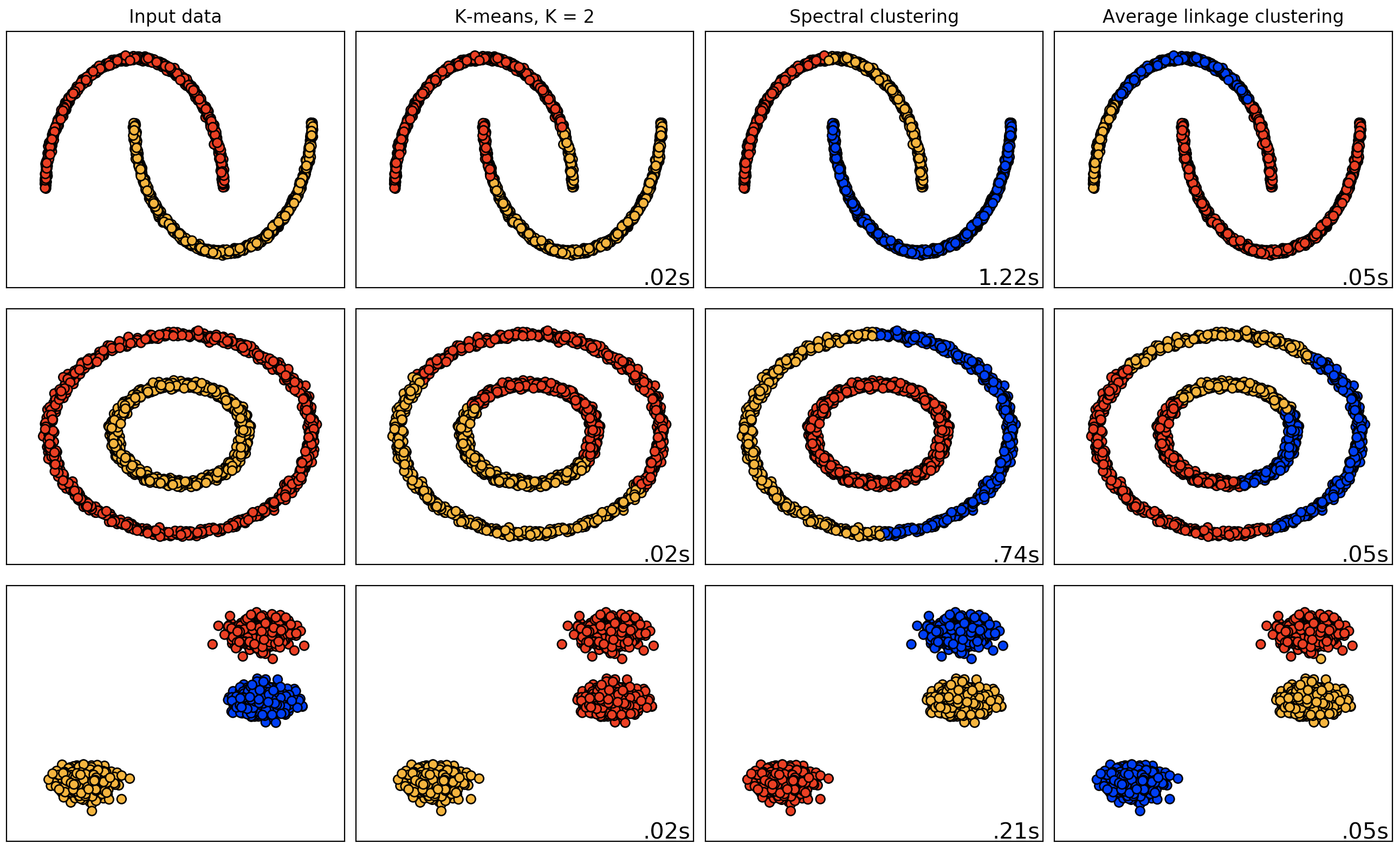## Exercises on machine learning

### Classification algorithms (supervised learning)

1. Read through `classifier_compare.py` and understand what is is doing.

2. Run the code by typing `python classifier_compare.py` into your terminal window. What do you observe? Comment on how each algorithm works on the different data sets. Why do some algorithms work better on some data sets than others? Also note the accuracy of each algorithm is specified in the bottom right corner of each plot.

3. Modify the code to test the following:

• K-nearest neighbor with K = 2
• K-nearest neighbor with K = 20
• Support vector machine with a sigmoid kernel function
• Support vector machine with a 3rd degree polynomial Comment on the results.
1. Python has an interesting dataset containing information about 3 different types of irises’ (Setosa, Versicolour, and Virginica) petal and sepal length. The code for importing this data and extracting the first two features is:
``````iris = datasets.load_iris()
X = iris.data[:, :2]
y = iris.target
``````

Apply a support vector machine and k-means classifier to this new data set. Vary the kernels and parameters to find the best classifier for this data set.

1. Sklearn also gives us the option to use custom-made kernels. We can do this using the following code as an example:
``````def my_kernel(X, Y):
K = np.dot(X, Y.T)
return K
clf = svm.SVC(kernel=my_kernel)
``````

Come up with your own custom kernel and apply it to a data set of your choice. How does your kernel compare to the other built-in kernels applied to this data?

### Clustering algorithms (unsupervised learning)

1. Read through `cluster_compare.py` and understand what is is doing.

2. Run the code by typing `python cluster_compare.py` into your terminal window. What do you observe? Comment on how each algorithm works on the different data sets. Why do some algorithms work better on some data sets than others? Also note the computation time of each algorithm is specified in the bottom right corner of each plot.

3. Increase the noise in the construction of the moon and circle data sets and re-run the code. What do you notice?

4. Change the number of clusters (or K for K-means) to 3. Re-run the code. What happens?

5. While K-means is efficient, Mini-Batch K-Means clustering is an even faster implementation of k-means. Modify cluster_compare.py to also appy Mini-Batch K-Means to the data-sets. The syntax for initiating this algorithm is:

``````cluster.MiniBatchKMeans(n_clusters=2)
``````

How do the computation times of k-means and Mini-batch k-means compare? What if you increase the size of the data set from 1500 to 5000? How do the kmeans algorithms compare to spectral clustering and average linkage clustering in terms of computation time?

1. As above, apply clustering algorithms of your choice to the first two features of Python’s iris data set. Comment on the results.

2. As we can see, having to know the number of clusters in your dataset is restrictive. DBSCAN is a clustering algorithm that does not require you to specify the number of clusters in your data–it finds them for you! All you have to specify is the maximum distance (epsilon) between two samples in order for them to be in the same cluster. Try applying DBSCAN to the three datasets in this code for varying values of epsilon using

``````cluster.DBSCAN(eps=epsilon)
``````

Can you find an epsilon that identifies the correct number of clusters across all data sets?

# Topological feature vectors - Euler curves

Slides can be found here.

This section contains python code to use the SECT (Smooth Euler Characteristic Transform) to compare images. For some theoretical results on the SECT, please see the paper Functional data analysis using a topological summary statistic: The smooth Euler characteristic transform at (https://arxiv.org/pdf/1611.06818.pdf) by Crawford, Monod, Chen, Mukherjee, and Rabadan.

## MNIST Example

MNIST is a dataset of hand-written digits. The following image is from wikipedia (https://en.wikipedia.org/wiki/MNIST_database).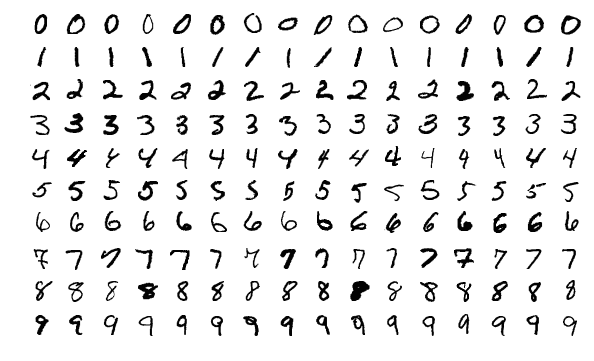At the webpage https://pjreddie.com/projects/mnist-in-csv/, download the MNIST training and testing csv files, which will be titled `mnist_test.csv` and `mnist_train.csv`. Copy these files into the `Top-ML/topological-feature-vectors/euler-curves` folder.

Change directories to this same `euler-curves` folder. In your terminal, try running the command

``````python mnist.py
``````

To get the above command to work, you first may need to install the progressbar package, perhaps via the command `pip install progressbar` (potentially with a sudo out front, if you are daring, depending on your permissions). This command takes around two minutes to run. You should git output like the following.

``````Generating EC for Test Set
Run: 100% |||||||||||||||||||||||||||||||||||||||||||||||||| Time: 0:00:05  17.79  B/s
Complete!
Generating EC for Train Set
Run: 100% |||||||||||||||||||||||||||||||||||||||||||||||||| Time: 0:00:12  16.61  B/s
Complete!
Computing mutual distances...
Run: 100% |||||||||||||||||||||||||||||||||||||||||||||||||| Time: 0:00:12   7.83  B/s
Complete!
Generating labels...
Run: 100% |||||||||||||||||||||||||||||||||||||||||||||||||| Time: 0:00:00   4.58 kB/s
Complete!
Accuracy on the test set: 86%
``````

This code computes the Euler characteristic curves for three directions on the MNIST training and testing data, using 200 training images and 100 testing images. It then predicts a label on the test data using K-nearest-neighbor, where distances are computed between Euler characteristic curves. This model identifies the digit in the test set with (around) 86% accuracy. It is possible to improve this to over 90% accuracy by increasing the training time and adding directions. It is important to note that this is nowhere near the state-of-the-art; it is not difficult to find classifiers on the MNIST data set that achieve an accuracy within a few percentage points of 100%!

## Devanagari Example

The following image of Devanagari script, which is used in India and Nepal, is from wikipedia (https://en.wikipedia.org/wiki/Devanagari). We will use a dataset with 36 different characters.We perform a similar classification task on the Devanagari script. At the webpage https://archive.ics.uci.edu/ml/datasets/Devanagari+Handwritten+Character+Dataset, download the folder at the link "Data Folder". The result should be called `DevanagariHandwritenCharacterDataset.zip`; unzip this folder and place it in the `Top-ML/topological-feature-vectors/euler-curves` folder.

In your terminal, try running the command

``````python devanagari.py
``````

To get the above command to work, you first may need to install the imageio and progressbar packages, perhaps via the commands `pip install imageio` and `pip install progressbar` (potentially with a sudo out front, if you are daring, depending on your permissions). This command takes around five minutes to run. You should git output like the following.

``````Generating EC for Test Set
Run: 100% |||||||||||||||||||||||||||||||||||||||||||||||||| Time: 0:00:36   5.00  B/s
Complete!
Generating EC for Train Set
Run: 100% |||||||||||||||||||||||||||||||||||||||||||||||||| Time: 0:00:58   6.13  B/s
Complete!
Computing mutual distances...
Run: 100% |||||||||||||||||||||||||||||||||||||||||||||||||| Time: 0:00:14  12.84  B/s
Complete!
Generating labels...
Run: 100% |||||||||||||||||||||||||||||||||||||||||||||||||| Time: 0:00:00   3.32 kB/s
Complete!
Accuracy on the test set: 68.0%
``````

This code computes the Euler characteristic curves for 12 directions, 10 training images and 5 testing images per letter. It then predicts a label on the test data using K-nearest-neighbor, where distances are computed between Euler characteristic curves. This model identifies the character in the test set with (around) 68% accuracy. This is fairly good on a classification task with 36 classes (36 different characters), as random guessing would give under 3% accuracy. It is possible to improve our accuracy to over 80% accuracy by increasing the training time.

# Persistent homology

For Henry's "introduction to persistent homology" slides from the ICERM TRIPODS bootcamp, please see http://www.math.colostate.edu/~adams/talks/AnIntroductionToAppliedAndComputationalTopology.pdf

We will be using Ripser (https://github.com/Ripser/ripser) in this tutorial. Some slides about how Ripser works are available at http://ulrich-bauer.org/ripser-talk.pdf.

## Ripser in your browser - synthetic examples

The easiest way to run Ripser is in a live demo in your browser, for which no instalation (and in particular, no Python) is required. Try it out! The webpage is http://live.ripser.org/.

### House example - distance matrix

For example, we can use Ripser to compute the persistent homology of the Vietoris-Rips complex of the following 5 points in the plane.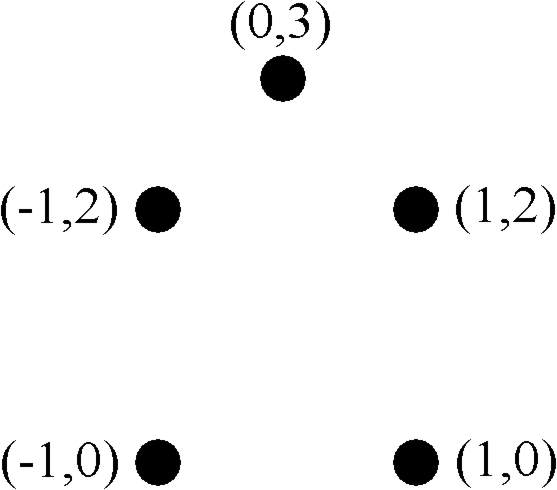The file house_distances.txt is in the folder `Top-ML/persistent-homology/distance_matrices`. It is the 5x5 distance matrix corresponding to the following collection of 5 points in the plane; the entries of this file are

``````0, 2.0000, 2.8284, 2.0000, 3.1623
2.0000, 0, 2.0000, 2.8284, 3.1623
2.8284, 2.0000, 0, 2.0000, 1.4142
2.0000, 2.8482, 2.0000, 0, 1.4142
3.1623, 3.1623, 1.4142, 1.4142, 0
``````

At the Ripser live webpage, enter the following input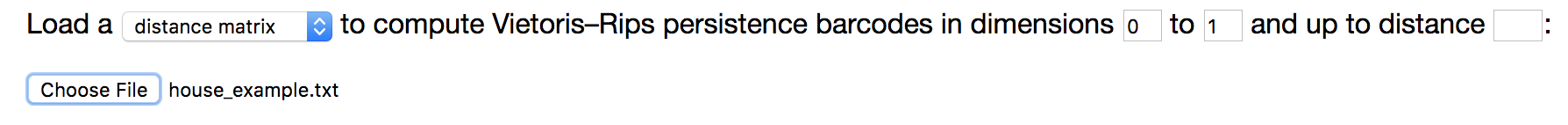Ripser should give the following output in your browser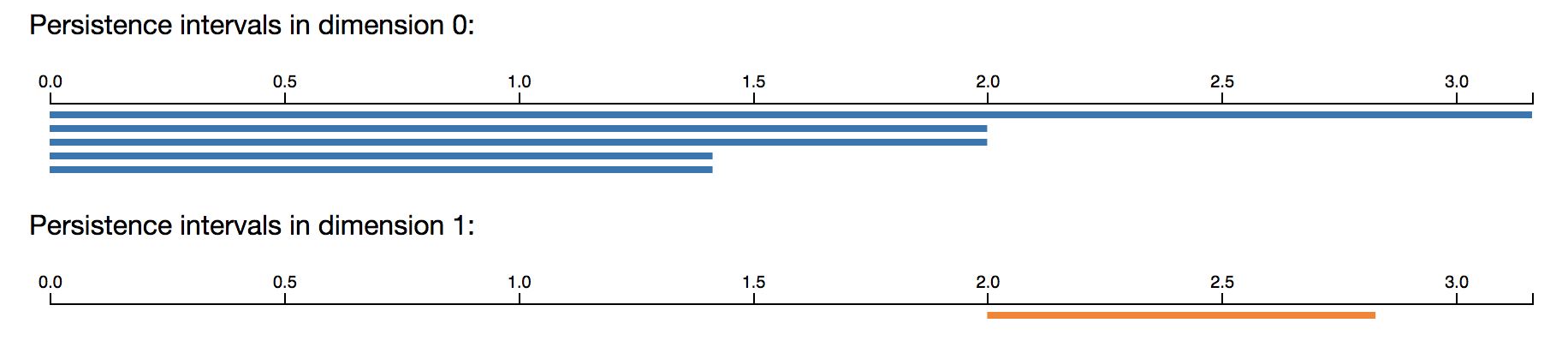Note that the 5 connected components merge into one, with merging events happening at scales (the square root of 2) and 2. There is a single 1-dimensional feature, beginning at scale parameter 2 and ending at scale parameter (the square root of 8).

### House example - point cloud

Instead of loading a distance matrix, it is also possible to to load in a set of points in Euclidean space, listed by their Euclidean coordinates. See for example the file `house_points.txt` in the folder `Top-ML/persistent-homology/point_clouds`. It is the 5x2 matrix corresponding to the same collection of 5 points in the plane. To input a point cloud in Ripser live, simply select the option `point cloud` instead of `distance matrix`. You will get the same output as above!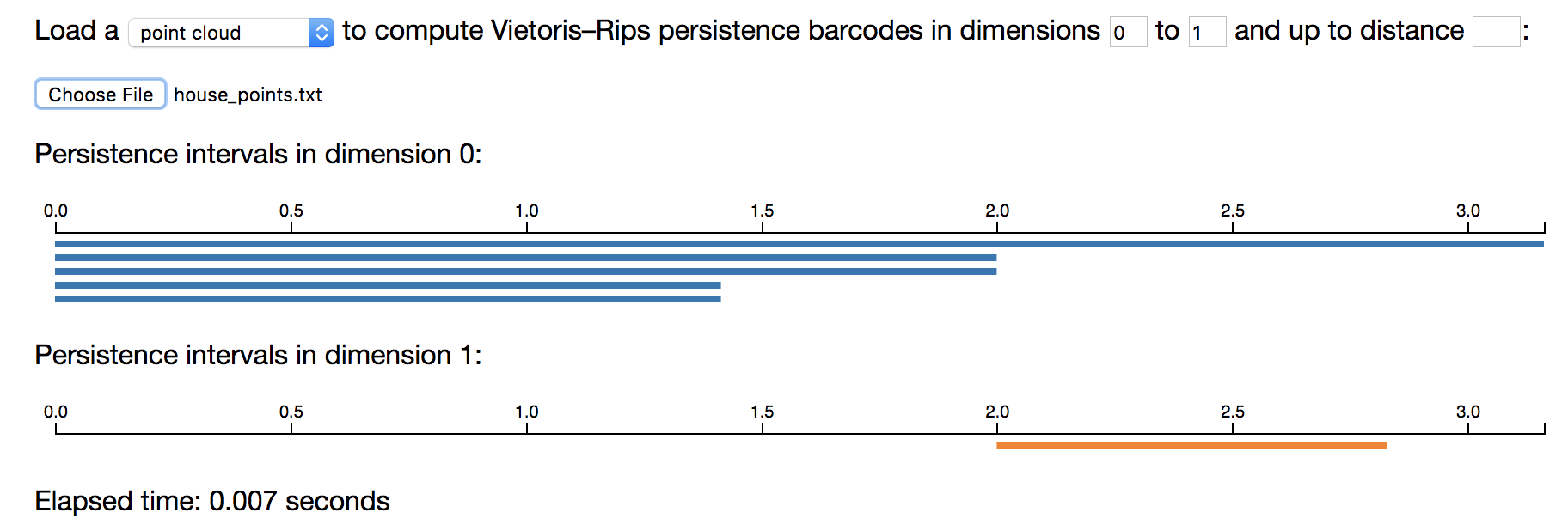### Torus example

The following example computes the persistent homology barcodes of a 20 x 20 grid of 400 points on the unit torus S1 x S1 in R4, where a small amount of noise has been added to each point. Only a subset of the intervals are shown below.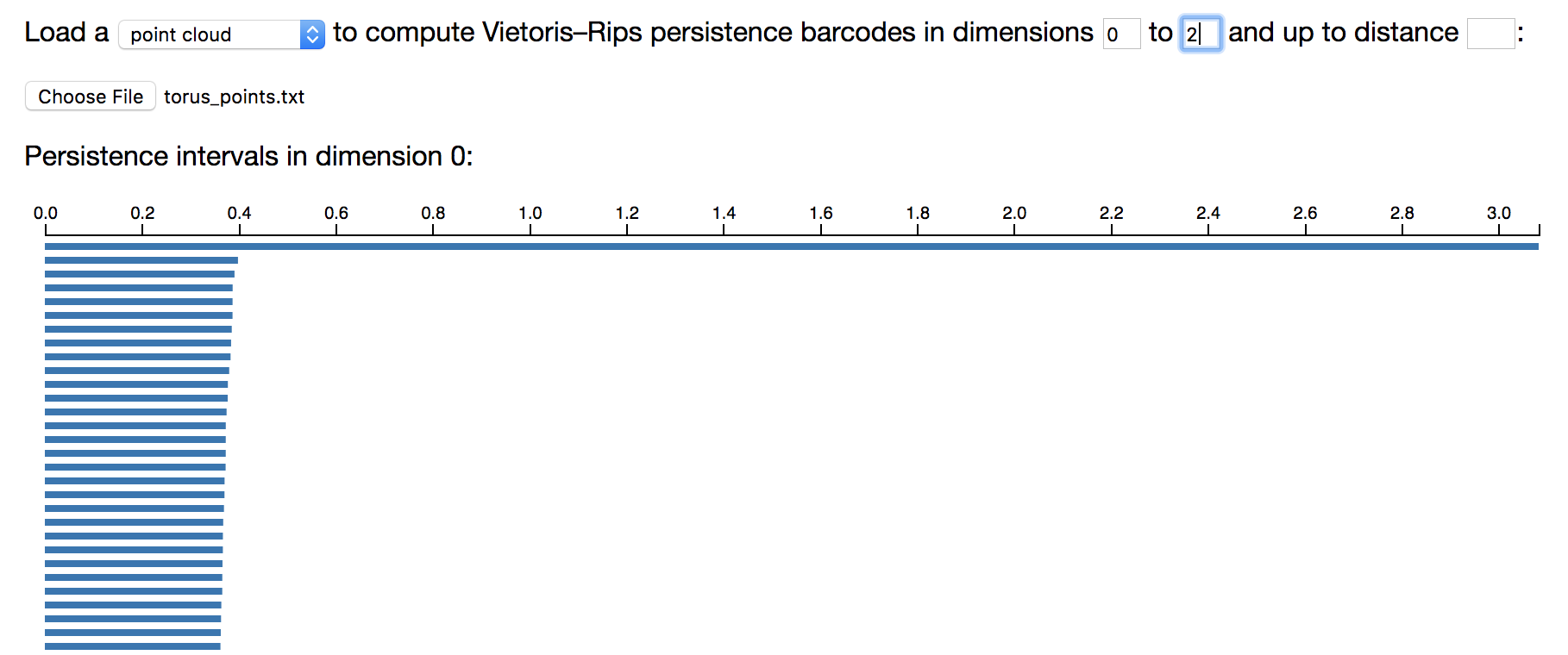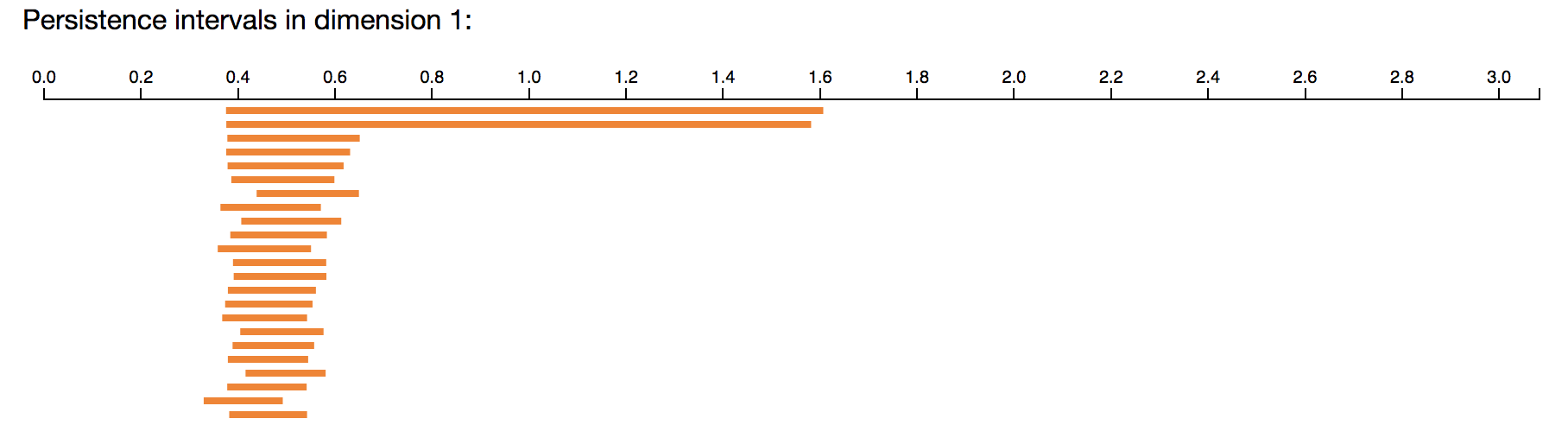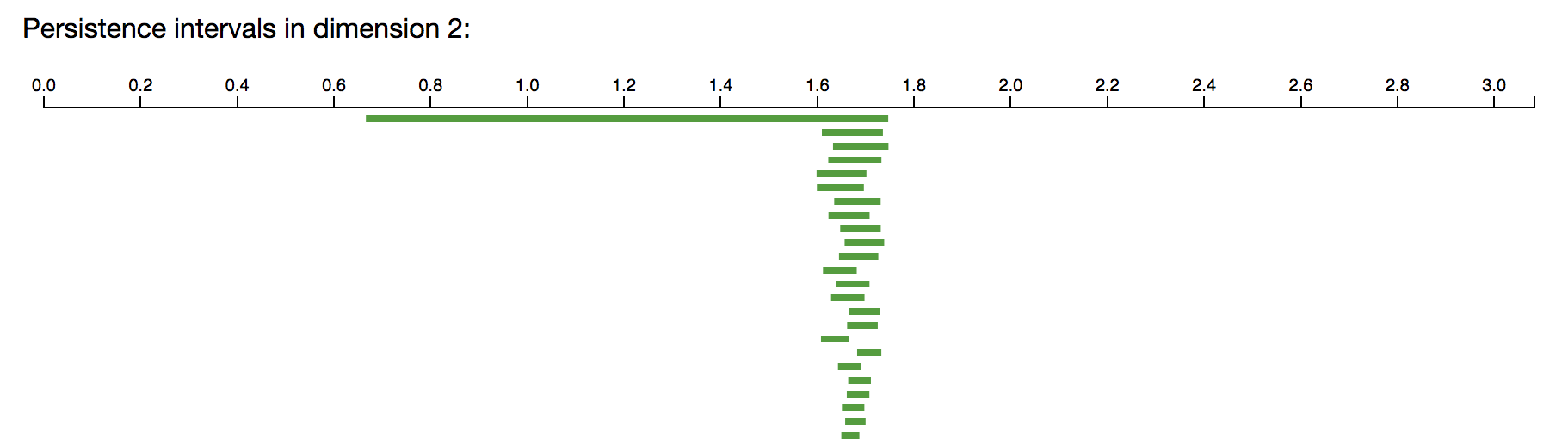Note that the long barcodes (roughly between scale parameter 0.7 and 1.5) recover the homology of the torus, with a single connected component, with two 1-dimensional holes, and with a single 2-dimensional hole.

### Sphere example, with a python script to create the points

Change directory to `Top-ML/persistent-homology`. Enter the following command into the terminal.

``````python sphere_points.py
``````

This is a Python script that samples 500 points from the unit sphere S2 in R3, and then saves the point cloud to the text file `sphere_points.txt` in the folder `point_clouds`.

(If you don't have Python running yet, the file `sphere_points.txt` should already be present in your folder `point_clouds`, and so you can just start from this step and feed those points into Ripser.)

We can compute the persistent homology barcodes in Ripser. So that the computation finishes, we have asked Ripser to compute only up to scale parameter 1.2 (one can compute larger examples after downloading Ripser to one's machine, as in a later section). Only a subset of the intervals are shown below.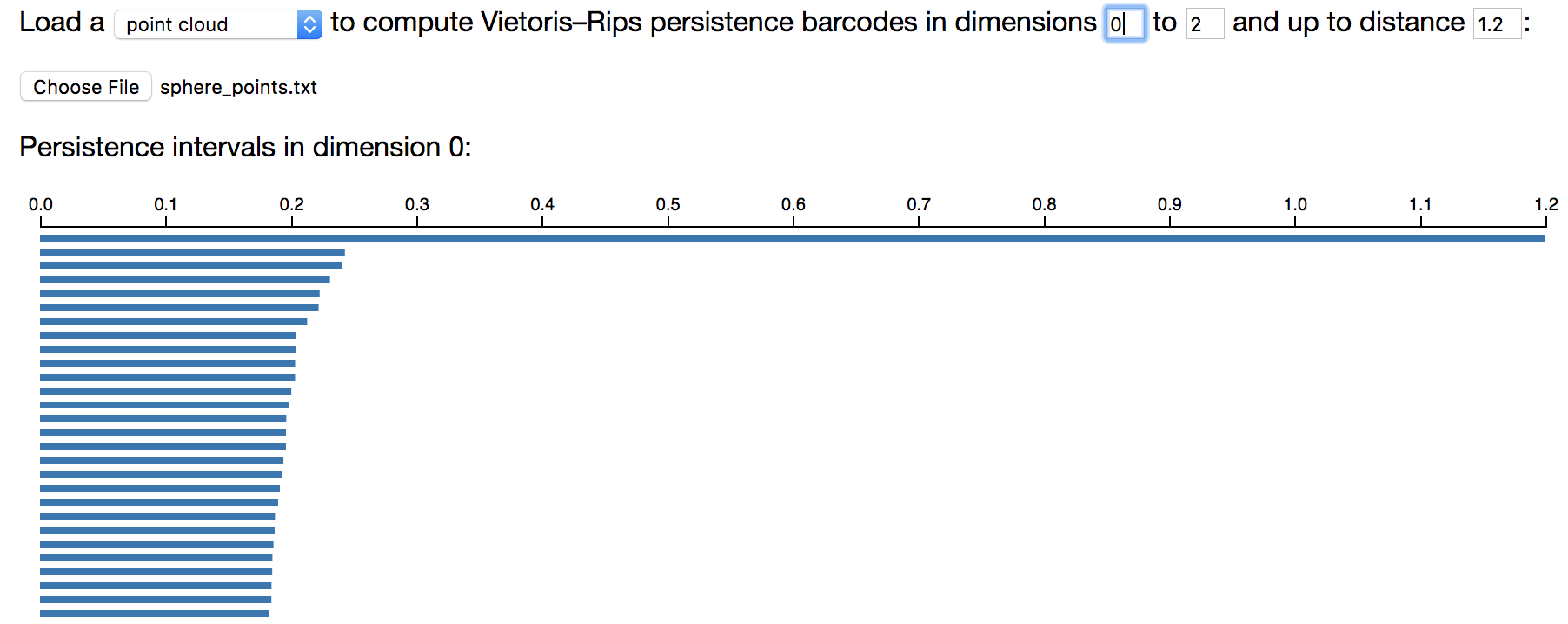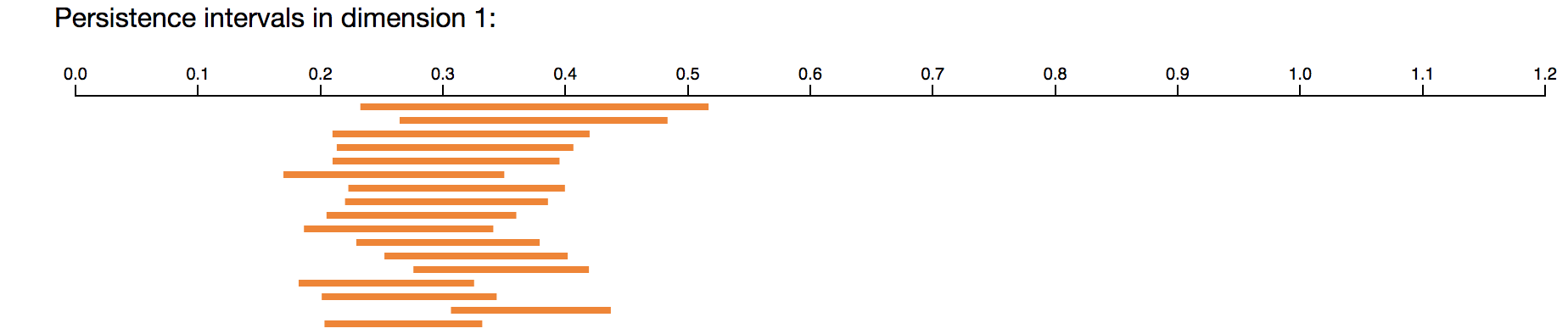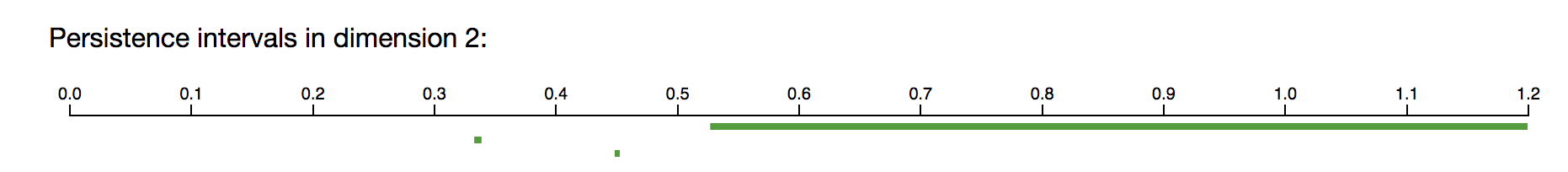Note that the long barcodes (roughly between scale parameter 0.55 and 1.2) recover the homology of the 2-sphere, with a single connected component, with no 1-dimensional holes, and with a single 2-dimensional hole.

## Ripser in your browser - cyclooctane data

This is an example with a real dataset of cyclooctane molecule conformations.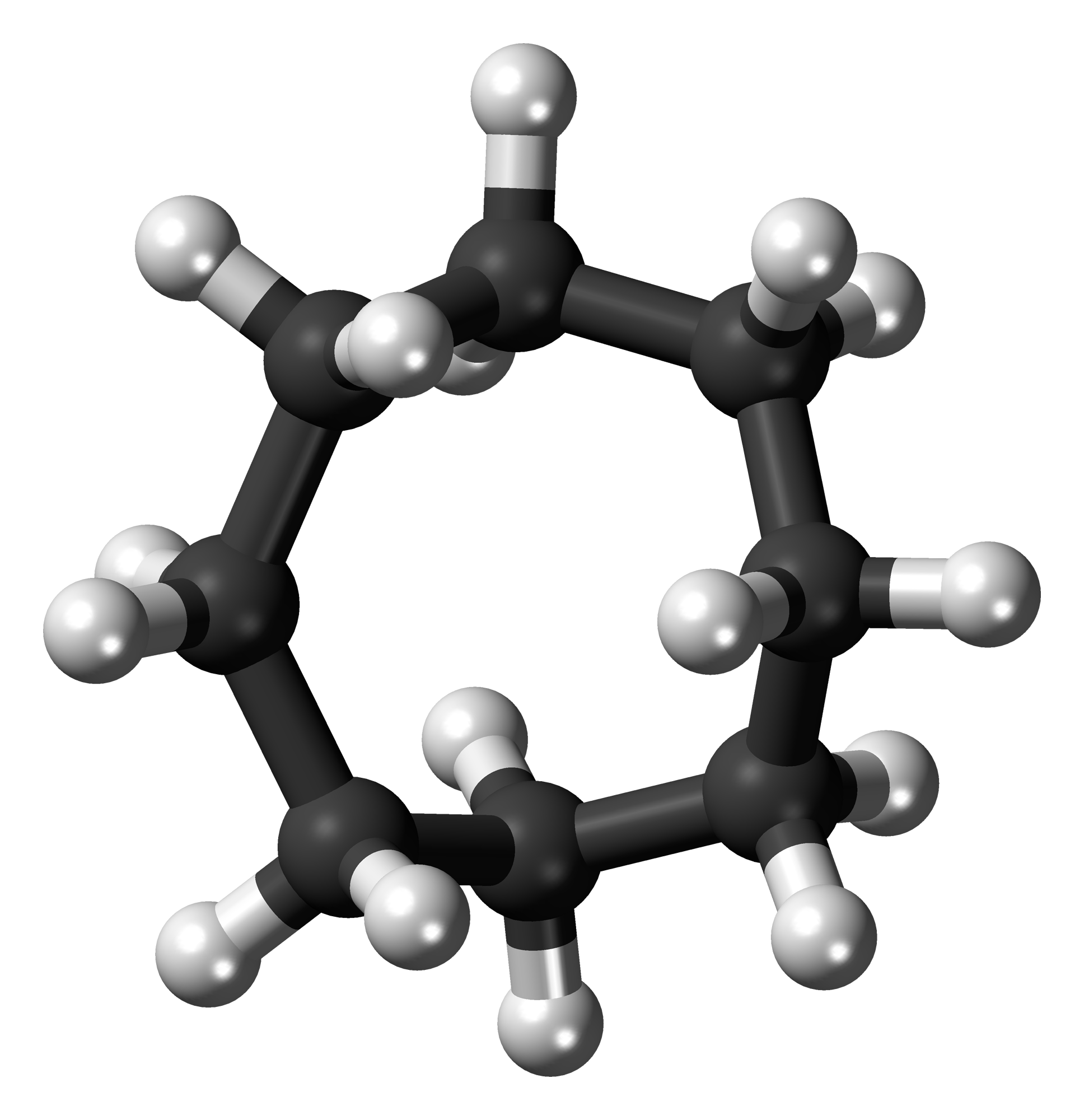The cyclooctane molecule consists of a ring of 8 carbon atoms (black), each bonded to a pair of hydrogen atoms (white).

The cyclooctane molecule C8H16 consists of a ring of 8 carbons atoms, each bonded to a pair of hydrogen atoms. A conformation of this molecule is a chemically and physically possible realization in 3D space, modulo translations and rotations. The locations of the carbon atoms in a conformation determine the locations of the hydrogen atoms via energy minimization, and hence each molecule conformation can be mapped to a point in R24=R8x3, as there are eight carbon atoms in the molecule and each carbon location is represented by three coordinates x,y,z. This map realizes the conformation space of cyclooctane as a subset of R24. It turns out that the conformation space is a two-dimensional stratefied space, i.e. a two-dimensional manifold with singularities. Furthermore, Brown et al. (2008), Martin et al. (2010), and Martin and Watson (2011) show that the conformation space of cyclooctane is the union of a sphere with a Klein bottle, glued together along two circles of singularities (see Figures 7 and 8 in Martin and Watson (2011)). Indeed, the algorithm they develop allows them to triangulate this conformation space from a finite sample.

Zomorodian (2012) uses the cyclooctane dataset as an example to show that we can efficiently recover the homology groups of the conformation space using persistent homology. In this section we essentially follow Zomorodian's example. We begin with a sample of 1,000 points on the conformation space (this data is publicly available at Shawn Martin's webpage http://www.sandia.gov/~smartin/software.html) and compute the resulting persistent homology. We obtain the Betti numbers Betti0 = Betti1 = 1 and Betti2 = 2, which match the homology groups of the union of a sphere with a Klein bottle, glued together along two circles of singularities.

The following example computes the persistent homology barcodes of 1,000 points from the configuration space of cyclooctane molecules in _R24. This dataset is in the file `Top-ML/persistent-homology/point_clouds/cyclooctane_points.txt`. So that the computation finishes, we have asked Ripser to compute only up to scale parameter 1.3 (one can compute larger examples after downloading Ripser to one's machine, as in a later section). Only a subset of the intervals are shown below.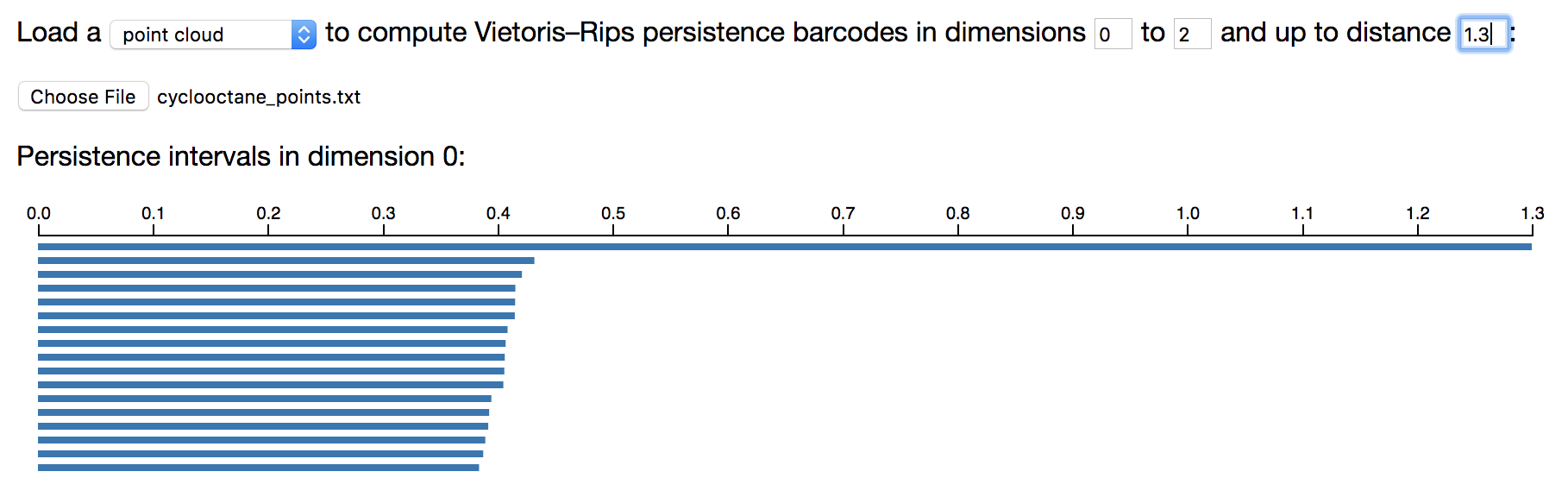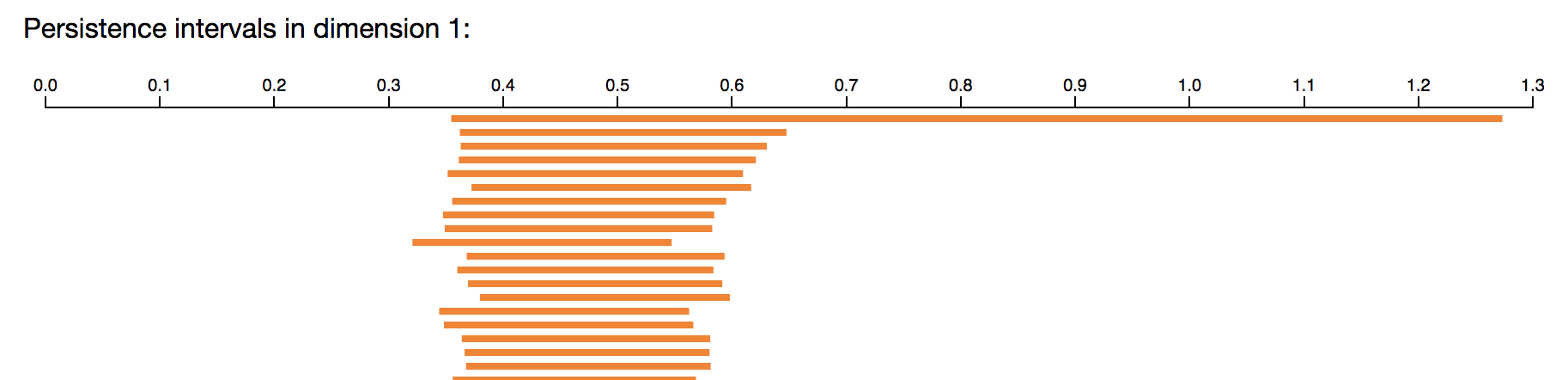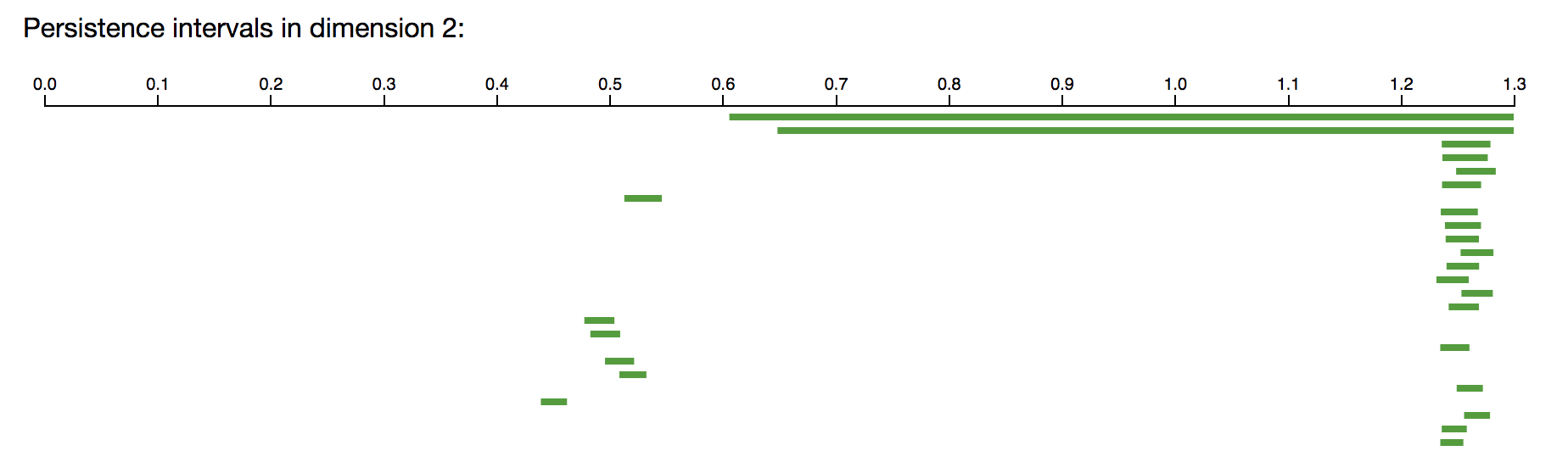The long barcodes (roughly between scale parameter 0.65 and 1.25), with one connected component, one 1-dimensional hole, and two 2-dimensional holes, match the homology of the union of a sphere with a Klein bottle, glued together along two circles of singularities.

## Ripser in your browser - optical image patch data

This is an example with a real dataset of optical image patch data.

The optical image database collected by van Hateren and van der Schaaf (1998) contains black and white digital photographs from a variety of indoor and outdoor scenes. Lee et al. (2003) study 3 x 3 patches from these images, and Carlsson et al. (2008) continue the analysis of image patches using persistent homology. Carlsson et al. (2008) begin with a large collection of high-contrast, normalized 3 x 3 pixel patches, each thought of as a point in R9. They change to the Discrete Cosine Transform (DCT) basis, which maps the patches to the unit sphere S7 in R8. They select from this space the 30% densest vectors, where density is based on the distance from a point to its 300th nearest neighbor. In Carlsson et al. (2008), this dense core subset is denoted X(300,30). In the next example we verify the result from Carlsson et al. (2008): X(300,30) has the topology of a circle.

The following example computes the persistent homology barcodes of 1,000 points from X(300,30). This dataset is in the file `Top-ML/persistent-homology/point_clouds/optical_k300_points.txt`. So that the computation finishes, we have asked Ripser to compute only up to scale parameter 1.3 (one can compute larger examples after downloading Ripser to one's machine, as in a later section). Only a subset of the intervals are shown below.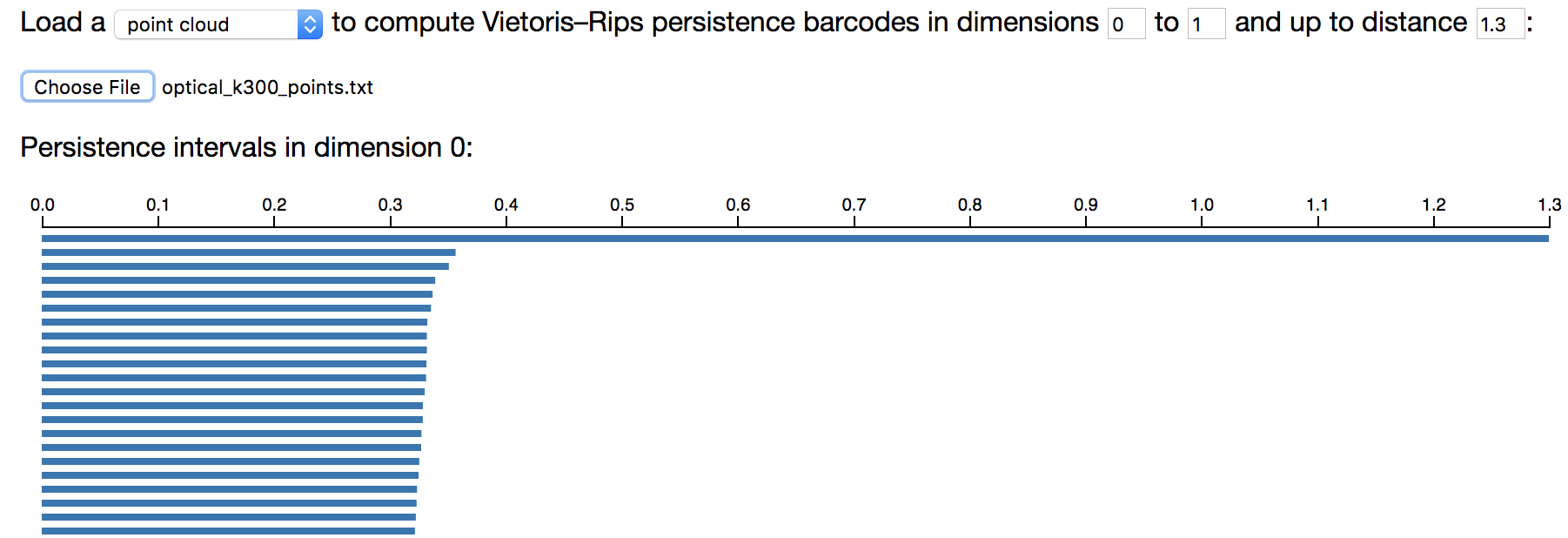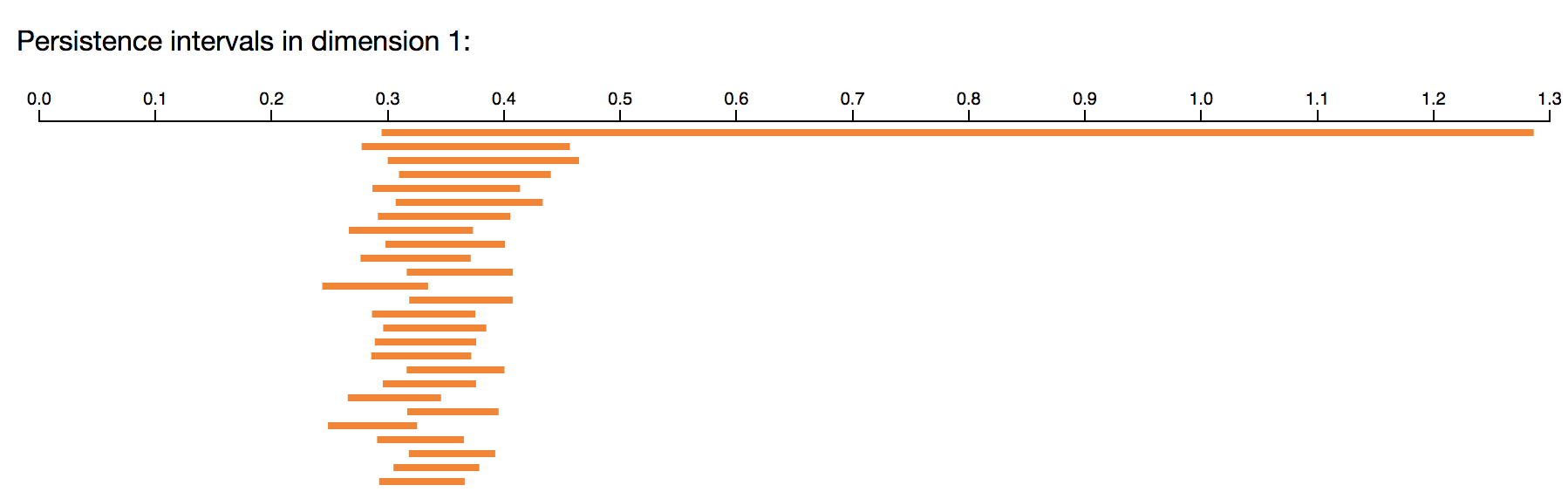The long barcodes (roughly between scale parameter 0.5 and 1.25), with one connected component and one 1-dimensional hole, have the homology of a circle. This is good evidence that the core subset X(300,30) is well-approximated by a circle.

We plot the projection of these points onto the first two linear gradient Discrete Cosine Transform basis vectors.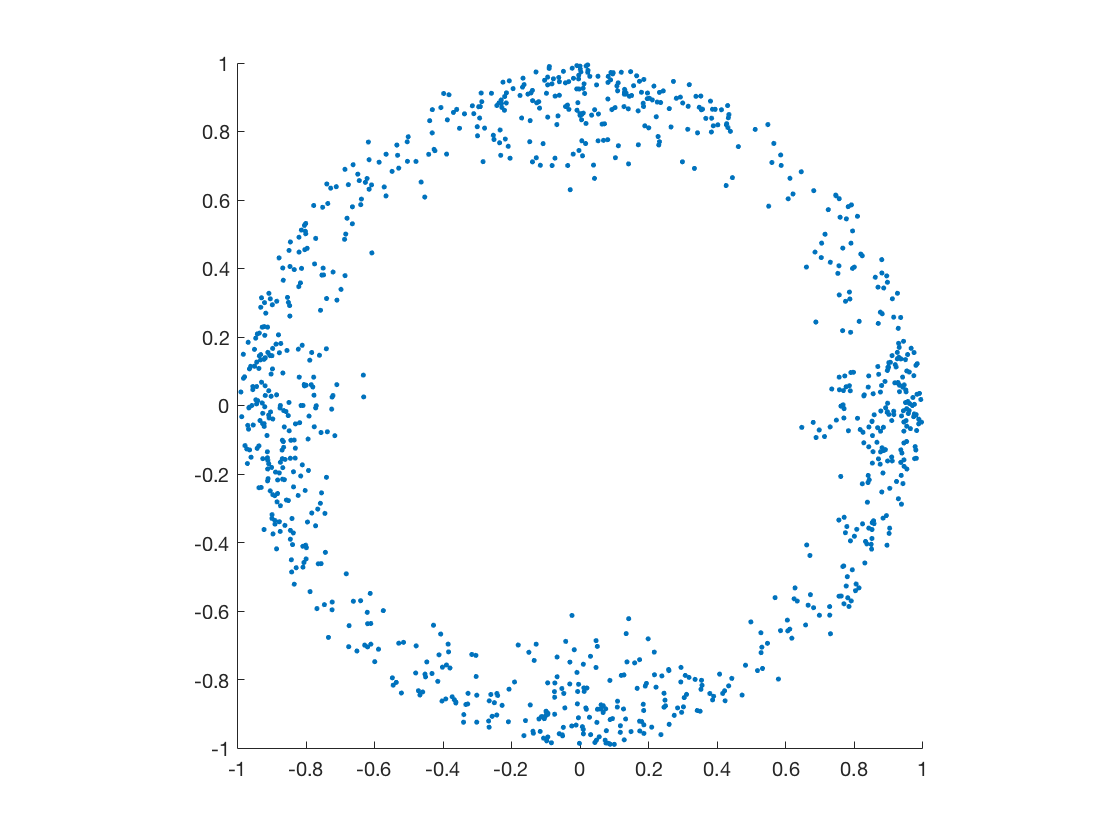Projection of X(300,30)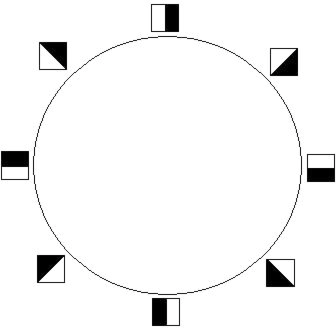Optical primary circle

The projection of X(300,30) above shows a circle. It is called the optical primary circle and is parameterized as shown above.

## Ripser on your machine

A more advanced (but very useful) step is to now download Ripser to your machine and to run it locally. This allows you to perform larger computations. Ripser is written in C++. You may download the code for Ripser at https://github.com/Ripser/ripser, which also contains installation instructions. Minimal installation instructions are listed below

``````git clone https://github.com/Ripser/ripser.git
cd ripser
make
./ripser examples/sphere_3_192.lower_distance_matrix
``````

For convenience, copy the Unix executable file `ripser` into the folder `Top-ML/persistent-homology`. You can use the flag `--format distances` to specify you are computing on a distance matrix, or `--format point-cloud` to specify you are computing on a point cloud. The flag `--dim k` specifies that homology is computed only up to dimension k, and the flag `--threshold t` specifies that persistent homology is computed only up to scale parameter t. For example, we can recreate all of the examples above with the following commands.

House example on the distance matrix:

``````./ripser --format distance distance_matrices/house_distances.txt
``````

House example on the point cloud:

``````./ripser --format point-cloud point_clouds/house_points.txt
``````

Torus example, up to 2-dimensional homology:

``````./ripser --format point-cloud --dim 2 point_clouds/torus_points.txt
``````

Sphere example, up to scale parameter 1.2:

``````./ripser --format point-cloud --dim 2 --threshold 1.2 point_clouds/sphere_points.txt
``````

Cyclooctane example. Try increasing the distance threshold gradually and see if your computer can do better than Ripser in your browser:

``````./ripser --format point-cloud --dim 2 --threshold 1.3 point_clouds/cyclooctane_points.txt
``````

Optical image patch example:

``````./ripser --format point-cloud --dim 1 --threshold 1.3 point_clouds/optical_k300_points.txt
``````

Instead of just printing the Ripser output to your screen, you can also save it to a text file. The below example also saves the Ripser output to the text file `house_points_ripser_printed.txt`.

``````./ripser --format point-cloud point_clouds/house_points.txt | tee -a house_points_ripser_printed.txt
``````

## Ripser with Python

Melissa Mcguirl has written very nice code for using Ripser with Python, which is what we will use in this section. In particular, the file `house_points_ripser_printed.txt` that we saved in the section above is not in a format that is terribly easy to work with. Melissa's code reformats Ripser output in a convenient manner!

Alternatively, there is a Cython wrapper for Ripser available which might be more efficient and better for non-Linux machines. The wrapper is available here: https://pypi.org/project/ripser/

Change directories to the `Top-ML/persistent-homology` folder. Copy the Ripser executable into this folder (or alternatively, make sure that ripser is in your Python path, and then in line 45 of `getBarCodes.py`, change "./ripser to "ripser"). In your terminal, try running the command

``````python getBarCodes.py -i distance_matrices/ -o ripser_outputs/
``````

This will take every distance matrix in the folder `distance_matrices`, compute the persistent homology barcodes for the Vietoris-Rips complex built on top of this metric space, and print the output barcodes to the folder `ripser_outputs`.

The command

``````python separateRipser.py -i ripser_outputs/ -o barcodes/
``````

then separates the ripser output into barcode intervals separated by dimension, and the command

``````python plotpd.py -i barcodes/ -o ./
``````

then plots the corresponding persistence diagrams in your current directory.

Melissa's code is only written to work with input metric space data in the form of a distance matrix, but one could edit it to also work with input metric space data in the form of a point cloud, for example.

## Exercises on persistent homology

Exercise: Write a script (say in Python, or some other language) that selects 500 points uniformly at random (or approximately uniformly at random) from the annulus {(x,y)|0.92≤x2+y2≤1.12} in R2. Compute its persistent homology barcodes using ripser.

Exercise: Write a script (say in Python, or some other language) that selects 500 points uniformly at random (or approximately uniformly at random) from the "coconut shell" {(x,y,z)|0.92≤x2+y2+z2≤1.12} in R3. Compute its persistent homology barcodes using ripser.

Exercise: Write a Python script (or code in some other language) that will select n even-spaced points from the unit circle in the plane. Compute the persistent homology of the Vietoris-Rips complex of 4 points, 6, 9, 12, 15 points, and 20 equally spaced points.

Do you get ever homology above dimension 1?

Exercise: Find a planar dataset Z in R2 and a filtration value t such that VR(Z,t) has nonzero Betti2. Do a computation in ripser to confirm your answer.

Exercise: Find a planar dataset Z in R2 and a filtration value t such that VR(Z,t) has nonzero Betti6. Do a computation in ripser to confirm your answer.

Exercise: Let X be the 8 vertices of the cube in R3: X={(±1, ±1, ±1)}. Equip X with the Euclidean metric. Compute the persistent homology of the Vietoris-Rips complex of X. Do you get ever homology above dimension 2?

Exercise: One way to produce a torus is to take a square [0, 1] x [0, 1] and then identify opposite sides. This is called a flat torus. More explicitly, the flat torus is the quotient space ([0, 1] x [0, 1]) / ~, where (0, y) ~ (1, y) for all y in [0, 1] and where (x, 0) ~ (x, 1) for all x in [0, 1]. The Euclidean metric on [0, 1] x [0, 1] induces a metric on the flat torus. For example, in the induced metric on the flat torus, the distance between (0, 1/2) and (1, 1/2) is zero, since these two points are identified. The distance between (1/10, 1/2) and (9/10, 1/2) is 2/10, by passing through the point (0, 1/2) ~ (1, 1/2).

Write a Python script (or code in another language) that selects 1,000 random points from the square [0, 1] x [0, 1] and then computes the 1,000 x 1,000 distance matrix for these points under the induced metric on the flat torus. Use Ripser to compute the persistent homology of this metric space.

Exercise: One way to produce a Klein bottle is to take a square [0, 1] x [0, 1] and then identify opposite edges, with the left and right sides identified with a twist. This is called a flat Klein bottle. More explicitly, the flat Klein bottle is the quotient space ([0, 1] x [0, 1]) / ~, where (0, y) ~ (1, 1 - y) for all y in [0, 1] and where (x, 0) ~ (x, 1) for all x in [0, 1]. The Euclidean metric on [0, 1] x [0, 1] induces a metric on the flat Klein bottle. For example, in the induced metric on the flat Klein bottle, the distance between (0, 4/10) and (1, 6/10) is zero, since these two points are identified. The distance between (1/10, 4/10) and (9/10, 6/10) is 2/10, by passing through the point (0, 4/10) ~ (1, 6/10).

Write a Python script (or code in another language) that selects 1,000 random points from the square [0, 1] x [0, 1] and then computes the 1,000 x 1,000 distance matrix for these points under the induced metric on the flat Klein bottle. Use Ripser to compute the persistent homology of this metric space.

Exercise: One way to produce a projective plane is to take the unit sphere S2 in R3 and then identify antipodal points. More explicitly, the projective plane is the quotient space S2 / (x ~ -x). The Euclidean metric on S2 induces a metric on the projective plane.

Write a Python script (or code in another language) that selects 1,000 random points from the unit sphere S2 in R3 and then computes the 1,000 x 1,000 distance matrix for these points under the induced metric on the projective plane. Use Ripser to compute the persistent homology of this metric space.

# Topological feature vectors - persistent homology and machine learning

There are by now a wide variety of ways to incorporate persistent homology information into feature vectors for a machine learning task (Adcock et al 2016, Adams et al 2017, Atienza 2018, Bendich et al 2016, Bubenik 2015, Bubenik & Dlotko 2016, Carriere et al 2015, Carriere & Bauer 2018, Carriere et al 2018, Chazal & Divol 2018, Chen et al 2015, Chevyrev et al 2018, Chung et al 2009, Di Fabio & Ferri 2015, Hofer et al 2017, Kalisnik 2018, Reininghaus et al 2015, Skraba 2018, Topaz et al 2015, Zeppelzauer et al 2016). We tersely describe a few of these approaches.

For Henry's "from persistent homology to machine learning" survey slides from the ICERM TRIPODS bootcamp, please see http://www.math.colostate.edu/~adams/talks/PHtoML_Slides.pdf

## Persistence landscpaes

One example is persistence landscapes (Bubenik 2015). Code is available (as described at Bubenik & Dlotko 2017) at https://www.math.upenn.edu/~dlotko/persistenceLandscape.html, and persistence landscapes are also implementable in R-TDA (https://rdrr.io/cran/TDA/man/landscape.html).

## Persistence images

A second example is persistence images (Adams et al 2017), with Python code available at https://github.com/scikit-tda/persim (Persims by Nathaniel Saul) or https://gitlab.com/csu-tda/PersistenceImages (by Francis Motta), and with Matlab code available at https://github.com/CSU-TDA/PersistenceImages.

We'll attempt to describe how to compute persistence images using the Jupyter notebooks accompanying Nathaniel Saul's Persim code (https://github.com/scikit-tda/persim). First, download the Persim code. Second, install Jupyter (http://jupyter.org/install.html), perhaps using Anaconda as described in that link. Open a new terminal (this is often required after new installations) and in that terminal, try the command `jupyter notebook` This should open a window in an internet browser. In this browser window, change directories to `persim/notebooks`, and then open the Jupyter notebook `Persistence Images.ipynb.` You should be able to run this code in the browser!

# Topological feature vectors - coding challenges

## 6 shape classes

Change directory to the folder `Top-ML/topological-feature-vectors/data-6-shape-classes`. This subfolder contains persistence diagram data for 6 shape classes: (1) A unit cube (2) A circle of diameter one (3) A sphere of diameter one (4) Three clusters with centers randomly chosen in the unit cube (5) Three clusters within three clusters (6) A torus with a major diameter of one and a minor diameter of one half. These shape classes are described in Section 6.1 of the paper "Persistence Images: A Stable Vector Representation of Persistent Homology"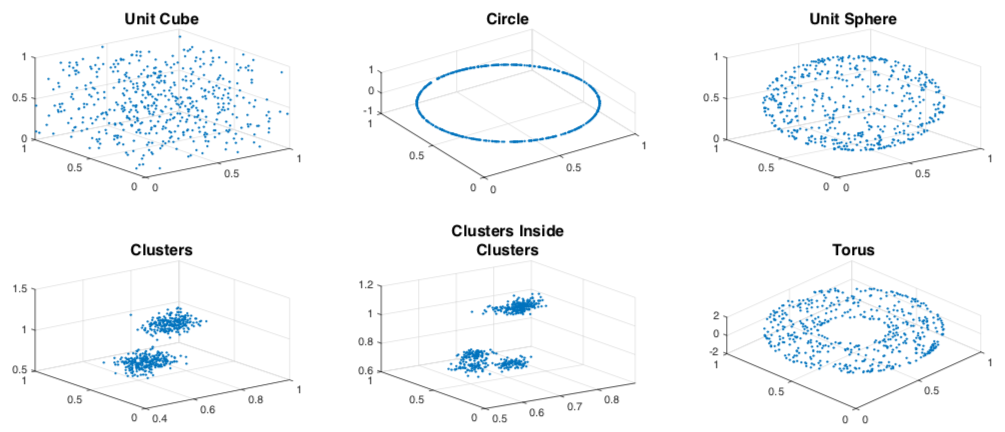We produced 25 point clouds of 500 randomly sampled points from each shape class. We then add a level of Gaussian noise to each point, at a noise level neta=0.1 or neta=0.05. We then have already computed the persistent homology intervals in homological dimension i=0 and i=1.

For example, the file ToyData_PD_n05_23_6_0.txt corresponds to noise level neta=0.05, the 23rd point cloud randomly sampled from shape class (6), with persistent homology computed in dimension 0. Each row of this file has two entries: the birth and death time of a 0-dimensional persistent homology interval.

By contrast, the file ToyData_PD_n1_21_3_1.txt corresponds to noise level neta=1, the 21st point cloud randomly sampled from shape class (3), with persistent homology computed in dimension 1. Each row of this file has two entries: the birth and death time of a 1-dimensional persistent homology interval.

Your task is to use machine learning to distinguish these six classes from each other. In a K-medoids clustering test, some accuracies and computation times are displayed for bottleneck distances, Wasserstein distances, persistence landscapes, and persistence images in Table 1 of "Persistence Images: A Stable Vector Representation of Persistent Homology". Do you have ideas for beating these accuracies or computation times?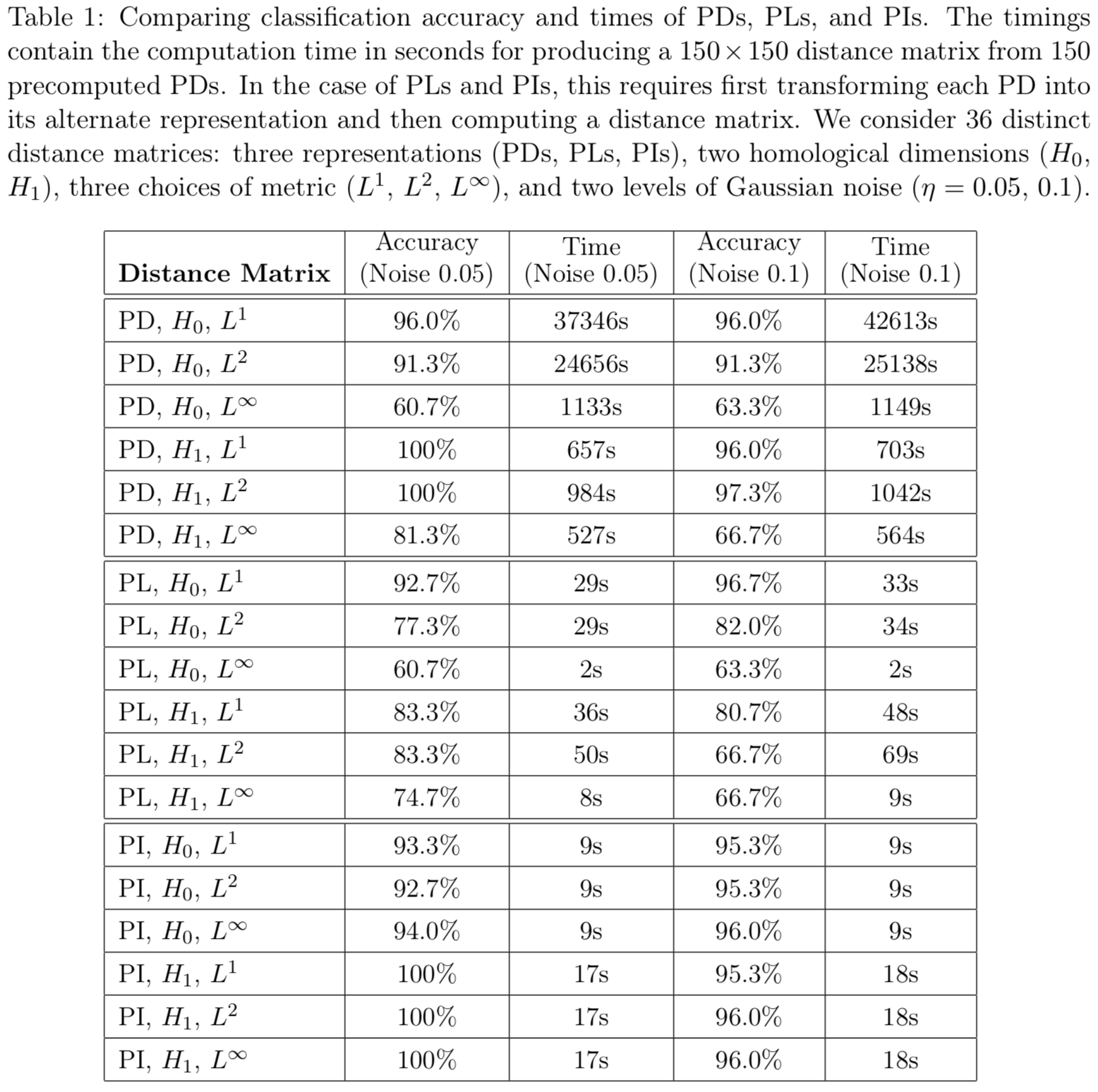In the above table, PD means the persistence diagram equipped with either the L1 (1-Wasserstein), L2 (2-Wasserstein), or sup (bottleneck) metrics. PL means the persistence landscape, equipped with either the L1, L2, or sup metric. PI means the persistence image, equipped with either the L1, L2, or sup metric. But you should develop your own techniques for turning barcodes into feature vectors, and see how they compare!

(Some Matlab code for creating points from these shape classes is available at https://github.com/CSU-TDA/PersistenceImages/tree/master/matlab_code/sixShapeClasses.)

## Other datasets to explore

Note, you may need to create a Kaggle account to access the data (it's free!).

Can we classify images?

Using SciPy, we can apply scipy.ndimage.imread (https://docs.scipy.org/doc/scipy-0.13.0/reference/generated/scipy.ndimage.imread.html) to read in image data into Python.

# Bibliography

A. Adcock, E. Carlsson, and G. Carlsson. The ring of algebraic functions on persistence bar codes. Homology, Homotopy and Applications, 18:381-402, 2016

H. Adams, S. Chepushtanova, T. Emerson, E. Hanson, M. Kirby, F. Motta, R. Neville, C. Peterson, P. Shipman, and L. Ziegelmeier. Persistence images: A stable vector representation of persistent homology. Journal of Machine Learning Research, 18:1-35, 2017.

P. Bendich, J.S. Marron, E. Miller, A. Pieloch, and S. Skwerer, Persistent homology analysis of brain artery trees. Ann. Appl. Stat., 10:198-218, 2016.

M. A. Armstrong. Basic Topology. Springer, New York, Berlin, 1983.

N. Atienza, R. Gonzalez-Diaz, and M. Soriano-Trigueros. On the stability of persistent entropy and new summary functions for topological data analysis. arXiv:1803.08304, 2018.

M. W. Brown, S. Martin, S. N. Pollock, E. A. Coutsias, and J. P. Watson. Algorithmic dimensionality reduction for molecular structure analysis. Journal of Chemical Physics, 129:064118, 2008.

P. Bubenik. Statistical topological data analysis using persistence landscapes. Journal of Machine Learning Research, 16:77-10, 2015.

P. Bubenik and P. Dlotko, A persistence lanscapes toolbox for topological statistics. Journal of Symbolic Computation, 78:91-114, 2017.

G. Carlson, T. Ishkhanov, V. de Silva, and A. Zomorodian. On the local behavior of spaces of natural images. Int. J. Computer Vision, 76:1-12, 2008.

M. Carriere, U. Bauer. On the metric distortion of embedding persistence diagrams into reproducing kernel Hilbert spaces. arXiv:1806.06924, 2018

M. Carriere, M. Cuturi, and S. Oudot. Sliced Wasserstein kernel for persistence diagrams. arXiv:1706.03358, 2017.

M. Carriere, S. Oudot, and M. Ovsjanikov. Stable topological signatures for points on 3d shapes. In Computer Graphics Forum, 34:1-2, 2015.

Y.-C. Chen, D. Wong, A. Rinaldo, and L. Wasserman. Statistical analysis of persistence intensity functions. arXiv:1510.02502, 2015.

L. Crawford, A. Monod, A. X. Chen, S. Mukherjee, and R. Rabadan. Functional data analysis using a topological summary statistic: The smooth Euler characteristic transform. arXiv: https://arxiv.org/abs/1611.06818, 2017.

F. Chazal, V. de Silva, and S. Oudot. Persistence stability for geometric complexes. Geometriae Dedicata, pages 1-22, 2013.

F. Chazal and V. Divol. The density of expected persistence diagrams and its kernel based estimation. arXiv:1802.10457, 2018.

I. Chevyrev, V. Nanda, H. Oberhauser. Persistence paths and signature features in topological data analysis. arXiv:1806.00381, 2018.

M.K. Chung, P. Bubenik, and P.T. Kim. Persistence diagrams of cortical surface data. In Information Processing in Medical Imaging, 386-297, Springer, 2009.

B. Di Fabio and M. Ferri. Comparing persistence diagrams through complex vectors. In International Conference on Image Analysis and Processing, LNCS 9279, pages 294-305, 2015.

H. Edelsbrunner and J. Harer. Computational Topology: An Introduction. American Mathematical Society, Providence, 2010.

H. Edelsbrunner, D. Letscher, and A. Zomorodian. Topological persistence and simplification. Discrete Comput. Geom., 28:511-533, 2002.

A. Hatcher. Algebraic Topology. Cambridge University Press, Cambridge, 2002.

C. Hofer, R. Kwit, M. Niethammer, and A. Uhl. Deep learning with topological signatures. In Advances in Neural Information Processing Systems, pages 1634-1644, 2017.

S. Kalisnik. Tropical coordinates on the space of persistence barcodes. In Foundations of Computational Mathematics, pages 1-29, 2018.

A. B. Lee, K. S. Pedersen, and D. Mumford. The nonlinear statistics of high-contrast patches in natural images. Int. J. Comput. Vision, 54:83-103, 2003.

S. Martin and J. P. Watson. Non-manifold surface reconstruction from high-dimensional point cloud data. Computational Geometry, 44:427-441, 2011.

S. Martin, A. Thompson, E. A. Coutsias, and J. P. Watson. Topology of cyclo-octane energy landscape. Journal of Chemical Physics, 132: 234115, 2010.

J. Reininghaus, S. Huber, U. Bauer, and R. Kwitt. A stable multi-scale kernel for topological machine learning. In Proceedings of the IEEE Conference on Computer Vision and Pattern Recognition, pages 4741-4748, 2015.

P. Skraba. Persistent homology and machine learning. Informatica, 24(2), 2018.

C. Topaz, L. Ziegelmeier, and T. Halverson. Topological data analysis of biological aggregation models. PloS One, 10(5):e0126383, 2015.

M. Zeppelzauer, B. Zielinski, M. Juda, and M. Seidl. Topological descriptors for 3d surface analysis. In Computational Topology in Image Context: 6th International Workshop Proceedings, pages 77-87, 2016.

J. H. van Hateren and A. van der Schaaf. Independent component filters of natural images compared with simple cells in primary visual cortex. Proc. R. Soc. Lond. B, 265:359-366, 1998.

A. Zomorodian. Advances in Applied and Computational Topology. American Mathematical Society, 2012.

A. Zomorodian and G. Carlsson. Computing persistent homology. Discrete Comput. Geom., 33:249-274, 2005.

##### Clone this wiki locally
You can’t perform that action at this time.